Click to Chat

1800-1023-196

+91-120-4616500

CART 0

• 0

MY CART (5)

Use Coupon: CART20 and get 20% off on all online Study Material

ITEM
DETAILS
MRP
DISCOUNT
FINAL PRICE
Total Price: Rs.

There are no items in this cart.
Continue Shopping• Complete Physics Course - Class 11
• OFFERED PRICE: Rs. 2,968
• View Details

Archimedes Principle

Table of Content

Refer this simulation for Archimedes Principle

Floatation

Related Resources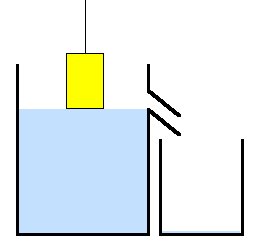When a body is partially or fully dipped into a fluid, the fluid exerts force on the body. At any small portion of the surface of the body, the force by the fluid is perpendicular to the surface and is equal to the product of the pressure at the surface and surface area. The resultant of all these contact forces is called buoyant force (upthrust).

Archimedes Principle states that "any body partially or completely submerged in a fluid is buoyed up by a force equal to the weight of the fluid displaced by the body."

Let a body is immersed in a fluid of density σ as shown in the figure.

F1 = A(h1 σg + p0) and F2 = A (h2 σg + p0)

As h2 > h1, hence F2 > F1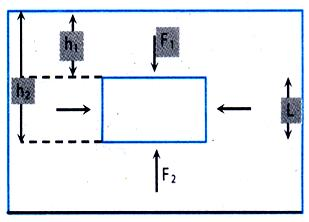So, the body will experience a net upward force.

F = F1 - F2 = Aσg (h2 - h1) = A gσL

= (AL) gσ =  V σg (V = volume of the body),

Hence, F = weight of fluid displaced by the body. This force is called buoyant force and acts vertically upwards (opposite to the weight of the body) through the centre of gravity of the displaced fluid.

Consider a stationary fluid in a container. Take a cylindrical volume of that fluid (as shown in figure) as a system.

Though this cylinder experiences the force of gravity due to its gravitational mass, it still remains stationary. This is so because the upward external force (P2A-P1A) balances the gravitational pull.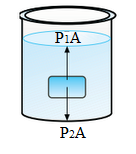A similar exercise can be carried out to show that the forces on the curved surface cancel each other. If an object is immersed in a fluid, it also experience an upward external force (P2A-P1A) which is known as the buoyant force. Whether a body floats or sinks, depends on the fact that whether the buoyant force is able to balance the weight or not.

Enquiry: Does buoyant force act only on an object, which is fully immersed in a fluid?

No, but for this, one has to understand the physical implication of buoyant force. If you recall, the buoyant force = (P2A-P1A)= (h2-h1)ρgA

Thus, we see that, the buoyant force is dependent on the volume of the immersed body. If a body is partly immersed, the thrust will only be on the lower surface and is equal to

ρgh.A   or ρgVsubmerged.Hence, it is evident that the buoyant force depends on the submerged volume of the body, be it partly or wholly immersed. You will note that the volume of the body immersed is same as the volume of the liquid displaced. Hence, in other words, we can say that the buoyant force depends on the volume of the fluid displaced.

Therefore, Buoyant force = Vρg where,  V = volume of fluid displaced, ρ = density of fluid

Or, Buoyant force  = weight of fluid displaced.

This fact in Physics was first discovered by Archimedes and is hence known as Archimedes principle, which states:

"When a body is immersed wholly or partly in a fluid, the body experiences a buoyant force equal to the weight of the fluid displaced by the body".

Question

If thrust on lower surface of an object immersed in a fluid is more, does the body experience a net force upwards?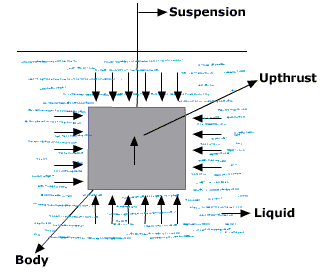Yes, a body immersed in a fluid does experience net upward force. To understand this let us first analyse a fluid element.

Question

Consider a container floating on water. Now we slowly pour water into it. The container goes down. Why?

Initially, force of buoyancy = weight of container.

Later, force of buoyancy = weight of container + weight of water in it

Hence, to increase the force of buoyancy the depth upto, which the cylinder is, submerged increase, as this will displace more water.

Apparent decrease in weight of the body = upthrust = weight of liquid displaced by the body

Refer this Simulation for Archimedes Principle

This animation shows the level a boat floats at when the density of the fluid is adjusted. You adjust the fluids density with a slider and the boat reacts.

Problem 1 (JEE Main)

The radii of the two columns is U-tube are r1 and r2. When a liquid density ρ (angle of contact is 0°) is filled in it, the level difference of liquid in two arms is h. Find out the surface tension of liquid.

Solution

We know that, h = 2T/rρg

h1 = 2T/r1ρg

and

h1 = 2T/r2ρg

h1 - h2 = h = 2T/ρg (1/r1 – 1/r2)

Thus, T = hρgr1r2/2(r2-r1)

Problem 2

A pipe of copper having an internal cavity weighs 264 gm in air and 221 gm in water. Find the volume of the cavity. [Density of copper is 8.8 gm/cc]

Solution

The buoyant force on the copper piece, F = Vrg

Hence, volume of the copper piece V = F/σg = (264-221)g/1xg = 43 cc

The volume of the material of the copper piece

V0 = mass of coper piece / density of material = 264/8.8 = 30 cc

Hence, volume of the cavity = V - V0 = 43 - 30 = 13 cc

Problem 3

A piece of brass (alloy of copper and zinc) weights 12.9 gm in air. When completely immersed in water, it weighs 11.3 gm. What is the mass of copper contained in the alloy?

Solution

Let the mass of copper in alloy = x gm.

So, Amount of zinc = (12.9 - x)gm

Volume of copper, VCu = x/ρCu = x/8.9 and

Volume of zinc VZn = (12.9-x)/7.1

So, Total volume of the alloy, V = VCu + VZn

or     V = (12.9-x)/7.1 + x/8.9                                                     ...... (i)

Buoyant force F = Vρ g = log of weight

= (12.9 - 11.3)g = 1.6 g

Substituting the value of V in equation (i), we get

x = 7.6 gm.

Problem 4

A hemispherical portion of radius R is removed from the bottom of a cylinder of radius R. The volume of the remaining cylinder is V and its mass is M. It is suspended by a string in a liquid of density r where it stays vertical. The upper surface of the cylinder is at a depth h below the liquid surface. Find the force on the bottom of the cylinder by the liquid.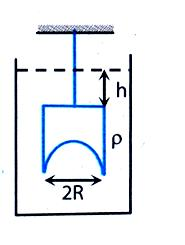Solution

Using Archimedes principle,

Fbottom - hρg(πR2) = Vrg

So, Fbottom = ρg(V + πR2h)

(ignoring atmospheric pressure)

Floatation

A body floats in a liquid if the average density of the body is less than that of the liquid. In such a case, the body is in equilibrium with a period of it immersed in the liquid.

(a)    The weight of the liquid displaced by the immersed part of the body must be equal to the weight of the body.

(b)    The centre of gravity of the body and centre of buoyancy must be along the same vertical line.

Enquiry: When does a body float?

If the buoyant force on a body equals its weight, the net force acting on the body becomes zero. Therefore, the body floats in equilibrium.

Let a body of total volume V & density d be floating on a liquid of density r. Let V1 be volume of the body inside the liquid.

Buoyant Force, B = mg

V1ρg = Vdg

or V1ρ = Vd

or V1/V = d/ρ.

When a body is immersed in a fluid of density r, the net downward force on it is

= mg-B

= Vdg - V1ρg.

This is also known as the apparent weight of the body.

When a body is immersed in a fluid, it feels lighter due to buoyant force. Hence buoyant force is also known as loss in weight i.e. (real weight-apparent weight).

Question

It is seen that in a balloon filled with air, if we press one side of the balloon, the other side bulges out. Why is it so?

If pressure at one point of liquid is increased, then pressure at all other points must also increase by same amount. This is so because pressure difference must remain the same between any two given points. Thus, the above phenomenon occurs. This law is known as Pascal's law. With this principle, lots of things have been simplified. For example, hydraulic lifts, usage of hydraulic brakes in cars, trucks etc.

Question

By using a hydraulic lift; how can lift a heavy car by applying a small force.

Consider the figure where there are two connected tubes of cross sectional area A1 & A2. Then, force required to lift mass m, F = A1/A2 mgIf A1 is less than A2, then F is also less than mg.

Hence, the concept used is that whatever excess pressure one applies to a fluid, this pressure gets transmitted to all other parts.

Think:  In the above illustration we are lifting up a much heavier object by applying a very small force. Is this not violating the law of conservation of energy.

Problem 5

A cube of wood supporting 200 gm mass just floats in water. When the mass is removed the cube rises by 2 cm. What is the size of the cube?

Solution:

Let the edge of the cube be x and the cube rises 2 cm on removing the mass. Thus, the weight of the body must be equal to the thrust provided by 2 cm height of the cube of base areas x2.

i.e. 200 g = (2 x2) 1 g

or x = 10 cm

Problem 6

A cubical block of iron of edge 5 cm floating on mercury in a vessel.

(a) What is the height of the block above mercury level?

(b) Water is poured into the vessel so that it just covers the iron block. What is the height of the water column?

[Relative density of Hg = 13.6 and that of Fe = 7.2]

Solution

(a)    Let h be the height of the iron block above mercury.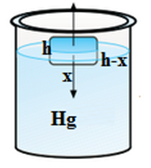In case of flotation,

Weight of the block = buoyant force

i.e., x3 ρ g = [(x - h) σ g]x2

Or h = x (1 -  ρ/σ) = 5(1 - 7.2/13.6)

= 2.35 cm.

(b)  Let y be the height of the water level.For equilibrium of the block,

x3 ρg = [σwgy + σHg g(x - y)]x2

xσ = (x - y) σHg + y σw

or y = x (σHg-σw)/(σHg-σw)

= 5 (13.6-7.2)/(13.6-1) = 2.54 cm

Problem 7

The 'tip of the iceberg' in popular speech has come to mean a small visible fraction of something that is mostly hidden. For real icebergs, what is the fraction? (ρice = 917 kg/m3, ρsea water = 1024 kg/m3)

Solution

Wice = ρi Vi g,      Wsea water = ρw Vw g

For floatation, ρi Vi g, = ρw Vw g

Fraction of the volume out of water = (Vi-Vw)/Vi

= 1024-917/1024 = 107/1024 = 10.45 %If the weight of the water displaced is less than the weight of the object, the object will sink.

If the weight of the water displaced is greater than the weight of the object the object will float, with the weight of the water displaced equal to the weight of the object.

This principle is useful for determining the volume and therefore the density of an irregularly shaped object by measuring its mass in air and its effective mass when submerged in water (density = 1 gram per cubic centimeter).

The buoyant force, which always opposes gravity, is nevertheless caused by gravity.

Fluid pressure increases with depth because of the (gravitational) weight of the fluid above.Question 1

What principle states that the buoyant force on an object in a fluid is an upward force equal to the weight of the fluid that the object displaces?

(a) Pascal’s Principle            (b) Archimedes’ Principle

(c) Newton's Principle            (d) Bernoulli’s Principle

Question 2

Fluid pressure is exerted evenly in ______ directions.

(a) all                           (b) downward

(c) side direction          (d) upward

Question 3

What is the upward force that keeps an object immersed in or floating on a liquid?

(a) pressure               (b) weight

(c) buoyant force       (d) density

Question 4

If an object’s weight is greater than the buoyant force, the object will ________.

(a) buoyed up        (b) sink

(c) do nothing        (d) float

Question 5

____________ pressure increases as depth increases.

(a) gas                  (b) liquid

(c) atmospheric     (d) waterQ.1
Q.2
Q.3
Q.4
Q.5

b

a

c

b

d

Related Resources:-

You might like to refer Fluid Statics.

For getting an idea of the type of questions asked, refer the  Previous Year Question Papers.### Course Features

• 101 Video Lectures
• Revision Notes
• Previous Year Papers
• Mind Map
• Study Planner
• NCERT Solutions
• Discussion Forum
• Test paper with Video Solution